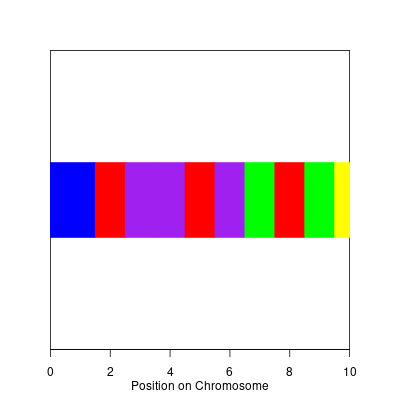I was asked over the weekend how to plot SNPs which are associated with specific ethnicities; the following code is a really quick stab at the problem using the grid graphics engine in R.

``````> require(grid)
> snp.position <- 1:10
> snp.integers <- sample.int(5,size=length(snp.position),replace=TRUE)
> ### the position is really the midpoint of the range
> snp.midpoint <- snp.position
> ### start of the SNP; we're assuming it should start at 0.
> snp.start <- c(0,snp.position[-1]-diff(snp.position)/2)
> ### stop of the snp; we're assuming it should stop at the last snp position
> snp.stop <- c(snp.position[-length(snp.position)]+diff(snp.position)/2,
>   snp.position[length(snp.position)])
> snp.width <- snp.stop - snp.start
>
> ### these are the colors
> possible.colors <- c("red","blue","yellow","green","purple")
> snp.colors <- possible.colors[snp.integers]
>
> ### this sets up the viewport that we'll plot into
> pushViewport(viewport(height=unit(1,"npc")-unit(7,"lines"),
> width=unit(1,"npc")-unit(7,"lines")
>   ))
> pushViewport(dataViewport(xscale=range(c(0,snp.stop)),yscale=c(0,1)))
>
> ### this draws a rectangle around the graph
> grid.rect()
> ### this sets up the x axis
> grid.xaxis()
> ### this labels the X axis
> grid.text("Position on Chromosome",y=unit(-2.5,"lines"))
>
> ### this draws all of the boxes corresponding to each SNP in the
> ### appropriate color
> grid.rect(x=unit(snp.start,"native"),
>         width=unit(snp.width,"native"),
>         y=unit(0.5,"native"),
>         height=unit(0.25,"native"),just=c("left","center"),
>         gp=gpar(col=snp.colors,fill=snp.colors))
>
> ### this pops the viewport
> popViewport(2)
``````